# 计算机浮点运算精度问题的一些探讨_薛梦冉

CSDN博客 · · 53 次点击 · · 开始浏览

##### 浮点运算常见的一些精度问题
``````相信各位在进行浮点型数据运算的时候,出现过一些不符合常规认知,或者是我们不愿出现的结果,

``````

mongoDB中对某种类型的交易金额聚合求和的时候:

``````db.FACT_TRADE_POSP.aggregate([
{\$match:{'AC_DT':'20200306','ETL_SOURCE':'05','TXN_CD':'2020060','TRAN_SOURCE':'03'}},
{\$group:{_id:null,total:{\$sum:1} ,mongo_txn:{\$sum:"\$TXN_AMT"}}}]);```

``````

``````{
"_id" : null,
"total" : 1594.0,
"mongo_txn" : 378808.66000000003
}

``````

Java浮点型运算:

`````` System.out.println(1.1 + 2.2);
``````

``````3.3000000000000003
``````

Python浮点型运算:

``````1.1 * 100
110.00000000000001
``````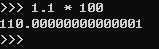##### 浮点运算精度问题产生的原因

###### 浮点运算精度问题的几点疑问
``````1. 所有的浮点运算都会产生精度问题么?
2. 对于存在精度问题的浮点运算来说,每次获取到的结果是恒定的还是随机的?
3. 不同的系统架构(例如:Java, Python)进行浮点运算产生的结果一致么?
``````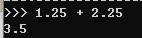``````// 在java中,用for循环打印1.1 + 2.2的运算结果
public static void main(String[] args) {
for (int i = 0; i < 10; i++) {
System.out.println(1.1 + 2.2);
}
}
``````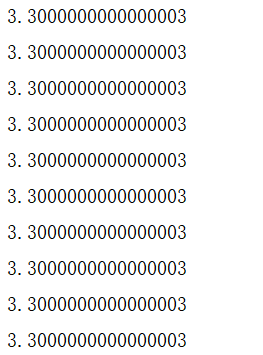(前提:针对的是相同的数据类型,并且输出打印显示的规则一致)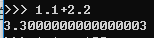###### 进制的相互转换

``````// 二进制转为十进制
10011 = 1 * 2^0 + 1 * 2^1 + 0 * 2^2 + 0 * 2^3 + 1 * 2^4 = 19
// 十进制转为二进制
19 / 2 = 9 .... 1
9 / 2 = 4 .... 1
4 / 2 = 2 .... 0
2 / 2 = 1 .... 0
1 / 2 = 0 .... 1
// 倒序排列 : 10011
``````

``````// 将未出现过精度问题的1.25转换为2进制
// 整数部分
1 / 2 = 0 .... 1
// 小数部分
0.25 * 2 = 0.5 .... 0
0.5 * 2 = 1.0 .... 1

// 将得到的二进制数1.01转化为10进制数
1 * 2^(-2) + 0 * 2^(-1) + 1 * 2^0 = 1.25

``````
``````
// 将出现过精度问题的1.1转为2进制
// 整数部分
1 / 2 = 0 .... 1
// 小数部分
0.1 * 2 = 0.2 .... 0
0.2 * 2 = 0.4 .... 0
0.4 * 2 = 0.8 .... 0
0.8 * 2 = 1.6 .... 1
0.6 * 2 = 1.2 .... 1
0.2 * 2 = 0.4 ---- 0
//这样,我们得到循环体是0011的无限循环,也就是说1.1永远无法精确的表现为二进制数
// 而我们实际做计算的时候,截取的只是其中的一部分,所以计算出来的结果一定会出问题
// 我们截取3个循环1.0001100110011看一下计算结果
1* 2^0 + 1*2^(-4) + 1*2^(-5) + 1 * 2^(-8) + 1 * 2 ^(-9) + 1 * 2 ^(-12) + 1 * 2^(-13)
= 1.0999755859375
// 可以看到和实际的1.1 还是存在差距的,当然,越往后面截取,这个差距就会越小
``````

###### 浮点数据的存储

(详情请参见下图:)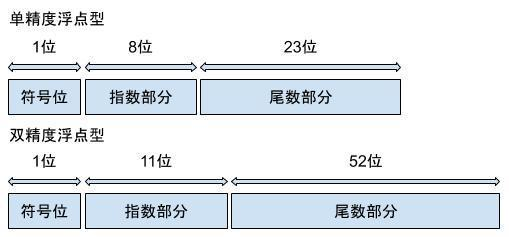为了便于计算和理解,通常我们采用正规化的浮点数进行运算

``````尾数不为0时，尾数域的最高有效位为1，这称为规格化。

浮点数x真值表示：
x=(−1)S×(1.M)×2e
``````

0是正数, 1是负数

128代表指数1, 126代表指数-1

``````// 第一步 将1.1转换为二进制
1.0001100110011001100110011001100110011001100110011001
(方括号里面表示的是小数点后52位及之后)
// 第二步 将得到的1.1的二进制正规化
1.0001100110011001100110011001100110011001100110011001
 * 2^0
-----------------------------
// 我们注意到:由于双精度浮点数尾数只有52位,因此需要进行舍入
/*
舍入规则如下:
若:第53位为1,且53位之后全部为0,此时就要使第52位变为0
若第52位本来就是0,不做处理
若52位本来为1,则第53位向前进一位,使第52位变为0

若53位为1, 且第53位之后不全为0,则第53位向第52位进一位完成向上舍入

若第53位为0,应该直接舍去不进位,称为下舍入
*/

1.0001100110011001100110011001100110011001100110011010 * 2^0
// 第三步 使用IEEE 754标准转换
S符号位 正数 符号位0
e 指数位

M尾数为:0001-1001-1001-1001-1001-1001-1001-1001-1001-1001-1001-1001-1010
``````

``````0 0111-1111-111 0001-1001-1001-1001-1001-1001-1001-1001-1001-1001-1001-1001-1010
``````

``````指数位为1023, 对应的实际指数为1

1.0001100110011001100110011001100110011001100110011010

``````

###### 浮点精度丢失问题的几种解决方案

1. 转换成整型进行计算

``````从我们最初二进制和十进制的转换中,我们知道,整型的转换不会出现问题,因此可以考虑将小数位数不多的数据转为整型计算

``````

2. 在业务没有进行精确要求的时候,可以采用四舍五入忽略这个问题

``````   其中:类似于大屏查询这种反馈整体情况的可以采用此方案
但是各种对精度要求很高的报表,对账单则需要慎重!
``````

Java中做法:

``````// 1. 直接使用Math.round(double)函数
Math.round(1.1 + 2.2) // 得到结果 : 3, 该方法返回值为long,会直接丢弃小数位,请慎用!
// 2. 方法1的改进方法,假设我要保留两位小数
(double)Math.round((1.1 + 2.2) * 100) /100
//得到结果3.3  并不是3.30哦,因为这里小数位数只有1位
``````

Python中做法:

``````# 使用round函数,返回浮点数的四舍五入值。
# 第二个参数为保留小数的位数,注意,若最后位的小数位为0,将不再保留,类似java
round(1.1 + 2.2, 1)
3.3
``````

3. 使用Decimal函数解决该问题

Java中做法:

``````// 1. 使用DecimalFormat进行计算
import java.text.DecimalFormat;
DecimalFormat dcmFmt = new DecimalFormat("0.00");
(Double.valueOf(dcmFmt.format(1.1 + 2.2)); //得到结果3.3
// 2. 使用BigDecimal进行计算
import java.math.BigDecimal;
``````

Python中做法:

`````` from decimal import Decimal
num = Decimal('1.1') + Decimal('2.2')
print(num) // 结果为3.3
``````
##### 参考资料

0 回复

• 请尽量让自己的回复能够对别人有帮助
• 支持 Markdown 格式, **粗体**、~~删除线~~、``单行代码``
• 支持 @ 本站用户；支持表情（输入 : 提示），见 Emoji cheat sheet
• 图片支持拖拽、截图粘贴等方式上传

##### 浮点运算常见的一些精度问题
``````相信各位在进行浮点型数据运算的时候,出现过一些不符合常规认知,或者是我们不愿出现的结果,

``````

mongoDB中对某种类型的交易金额聚合求和的时候:

``````db.FACT_TRADE_POSP.aggregate([
{\$match:{'AC_DT':'20200306','ETL_SOURCE':'05','TXN_CD':'2020060','TRAN_SOURCE':'03'}},
{\$group:{_id:null,total:{\$sum:1} ,mongo_txn:{\$sum:"\$TXN_AMT"}}}]);```

``````

``````{
"_id" : null,
"total" : 1594.0,
"mongo_txn" : 378808.66000000003
}

``````

Java浮点型运算:

`````` System.out.println(1.1 + 2.2);
``````

``````3.3000000000000003
``````

Python浮点型运算:

``````1.1 * 100
110.00000000000001
``````##### 浮点运算精度问题产生的原因

###### 浮点运算精度问题的几点疑问
``````1. 所有的浮点运算都会产生精度问题么?
2. 对于存在精度问题的浮点运算来说,每次获取到的结果是恒定的还是随机的?
3. 不同的系统架构(例如:Java, Python)进行浮点运算产生的结果一致么?
````````````// 在java中,用for循环打印1.1 + 2.2的运算结果
public static void main(String[] args) {
for (int i = 0; i < 10; i++) {
System.out.println(1.1 + 2.2);
}
}
``````(前提:针对的是相同的数据类型,并且输出打印显示的规则一致)###### 进制的相互转换

``````// 二进制转为十进制
10011 = 1 * 2^0 + 1 * 2^1 + 0 * 2^2 + 0 * 2^3 + 1 * 2^4 = 19
// 十进制转为二进制
19 / 2 = 9 .... 1
9 / 2 = 4 .... 1
4 / 2 = 2 .... 0
2 / 2 = 1 .... 0
1 / 2 = 0 .... 1
// 倒序排列 : 10011
``````

``````// 将未出现过精度问题的1.25转换为2进制
// 整数部分
1 / 2 = 0 .... 1
// 小数部分
0.25 * 2 = 0.5 .... 0
0.5 * 2 = 1.0 .... 1

// 将得到的二进制数1.01转化为10进制数
1 * 2^(-2) + 0 * 2^(-1) + 1 * 2^0 = 1.25

``````
``````
// 将出现过精度问题的1.1转为2进制
// 整数部分
1 / 2 = 0 .... 1
// 小数部分
0.1 * 2 = 0.2 .... 0
0.2 * 2 = 0.4 .... 0
0.4 * 2 = 0.8 .... 0
0.8 * 2 = 1.6 .... 1
0.6 * 2 = 1.2 .... 1
0.2 * 2 = 0.4 ---- 0
//这样,我们得到循环体是0011的无限循环,也就是说1.1永远无法精确的表现为二进制数
// 而我们实际做计算的时候,截取的只是其中的一部分,所以计算出来的结果一定会出问题
// 我们截取3个循环1.0001100110011看一下计算结果
1* 2^0 + 1*2^(-4) + 1*2^(-5) + 1 * 2^(-8) + 1 * 2 ^(-9) + 1 * 2 ^(-12) + 1 * 2^(-13)
= 1.0999755859375
// 可以看到和实际的1.1 还是存在差距的,当然,越往后面截取,这个差距就会越小
``````

###### 浮点数据的存储

(详情请参见下图:)为了便于计算和理解,通常我们采用正规化的浮点数进行运算

``````尾数不为0时，尾数域的最高有效位为1，这称为规格化。

浮点数x真值表示：
x=(−1)S×(1.M)×2e
``````

0是正数, 1是负数

128代表指数1, 126代表指数-1

``````// 第一步 将1.1转换为二进制
1.0001100110011001100110011001100110011001100110011001
(方括号里面表示的是小数点后52位及之后)
// 第二步 将得到的1.1的二进制正规化
1.0001100110011001100110011001100110011001100110011001
 * 2^0
-----------------------------
// 我们注意到:由于双精度浮点数尾数只有52位,因此需要进行舍入
/*
舍入规则如下:
若:第53位为1,且53位之后全部为0,此时就要使第52位变为0
若第52位本来就是0,不做处理
若52位本来为1,则第53位向前进一位,使第52位变为0

若53位为1, 且第53位之后不全为0,则第53位向第52位进一位完成向上舍入

若第53位为0,应该直接舍去不进位,称为下舍入
*/

1.0001100110011001100110011001100110011001100110011010 * 2^0
// 第三步 使用IEEE 754标准转换
S符号位 正数 符号位0
e 指数位

M尾数为:0001-1001-1001-1001-1001-1001-1001-1001-1001-1001-1001-1001-1010
``````

``````0 0111-1111-111 0001-1001-1001-1001-1001-1001-1001-1001-1001-1001-1001-1001-1010
``````

``````指数位为1023, 对应的实际指数为1

1.0001100110011001100110011001100110011001100110011010

``````

###### 浮点精度丢失问题的几种解决方案

1. 转换成整型进行计算

``````从我们最初二进制和十进制的转换中,我们知道,整型的转换不会出现问题,因此可以考虑将小数位数不多的数据转为整型计算

``````

2. 在业务没有进行精确要求的时候,可以采用四舍五入忽略这个问题

``````   其中:类似于大屏查询这种反馈整体情况的可以采用此方案
但是各种对精度要求很高的报表,对账单则需要慎重!
``````

Java中做法:

``````// 1. 直接使用Math.round(double)函数
Math.round(1.1 + 2.2) // 得到结果 : 3, 该方法返回值为long,会直接丢弃小数位,请慎用!
// 2. 方法1的改进方法,假设我要保留两位小数
(double)Math.round((1.1 + 2.2) * 100) /100
//得到结果3.3  并不是3.30哦,因为这里小数位数只有1位
``````

Python中做法:

``````# 使用round函数,返回浮点数的四舍五入值。
# 第二个参数为保留小数的位数,注意,若最后位的小数位为0,将不再保留,类似java
round(1.1 + 2.2, 1)
3.3
``````

3. 使用Decimal函数解决该问题

Java中做法:

``````// 1. 使用DecimalFormat进行计算
import java.text.DecimalFormat;
DecimalFormat dcmFmt = new DecimalFormat("0.00");
(Double.valueOf(dcmFmt.format(1.1 + 2.2)); //得到结果3.3
// 2. 使用BigDecimal进行计算
import java.math.BigDecimal;
`````` from decimal import Decimal